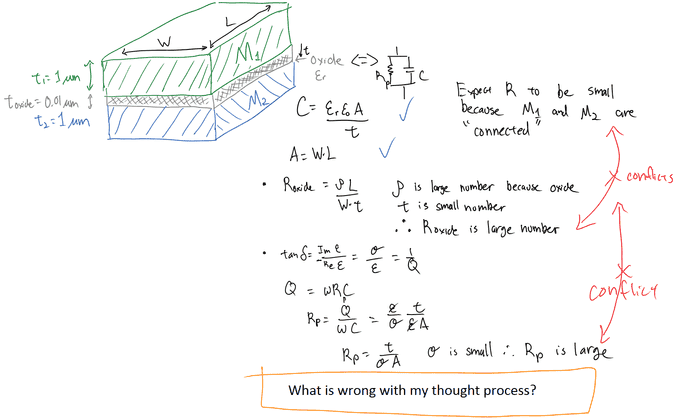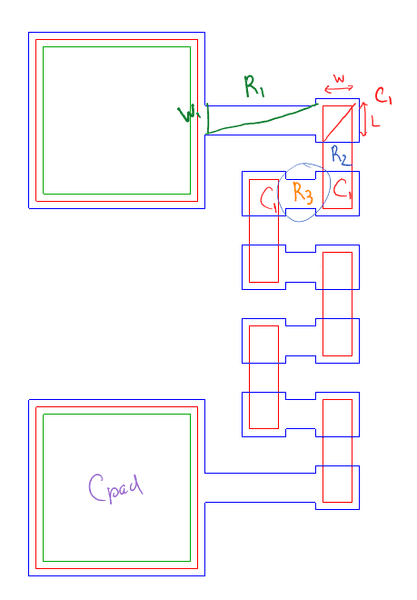# Resistance and Capacitance of Two Metal Plates "Touching"

## Homework Statement

Two conductive metal plates are bonded together. Upon bonding, an oxide layer forms between them. The oxide layer is negligible thus the overall resistance of the plates is small. However I am having trouble finding this overall resistance, i.e., trying to find Cp and Rp.

Dimensions and properties of M1,M2, and the oxide layer are known.
Oxide thickness << M1 and M2 thickness

## Homework Equations

Parallel plate capacitance equation: C = epsilon A / t
Wire resistance equation: R = rho L /A
Loss tangent of capacitor and quality factor to get the parallel resistance

## The Attempt at a Solution

The resistance of the oxide layer is high but how do I apply this to the overall resistance?

The attached image shows my work.Thanks gents.

rude man
Homework Helper
Gold Member
.
Rp is not going to be small. What do you mean, "should be small because the plates are connected"? They are NOT connected, they are isolated from each other by the oxide layer, however small.

You can find Rp and Cp as follows: apply a variable-frequency sine voltage between the plates. Measure the phase shift between the voltage and the current (use a sampling resistor Rs << 1/ωCp for the current). Increase the frequency ω until you get 45 degrees of phase shift between voltage and current. Then Rp = 1/ωCp and a second equation is current = voltage*√(Gp2 + ω2Cp2)
where Gp = 1/Rp. Solve for Rp and Cp.

rude man
Homework Helper
Gold Member
Additional note: picking Rs: approximate Cp = εA/t; you can look up ε for the oxide in a handbook; you just need an approximate number. Then when you adjust ω to get 45 degrees phase shift, check that Rs << 1/ωCp.

My guess is you'll find that Rp is so high that you'll need a very low ω which in turn means very low current amplitude, maybe too low to measure easily.

NascentOxygen
Staff Emeritus
SeriousNoob said:
Parallel plate capacitance equation: C = epsilon A / t
Wire resistance equation: R = rho L /A
Loss tangent of ê and quality factor to get the parallel resistance

I presume you are concerned with calculating these parameters, and are not doing an experiment to measure them.

Your formulae I quote are correct, but in your calculations you misapplied the formula for R; try that again. The resistance of the metal itself is negligible (i.e., close to 0 ohms), and the parallel resistance of the 'gap' is what you calculate as the bulk resistance of that oxide dielectric. Is its total thickness 0.01 or is it 0.02? Check that you have understood the question specification correctly.

I am simulating a structure and trying to model it as a daisy chain of lumped components.

The image below is the structure I am simulating. Red is M1 and Blue is M2. I have an oxide layer between M1 and M2, though not shown in the image. The numbers I showed above are arbitrary.

I say C1 has a low parallel resistance because my lumped model and simulation match better at low frequency when I have a small Rp value.I think of it as this way. When bonding metals together an unwanted oxide layer is form between them but the metals are very much conductive. Imaging a relatively "thick" oxide between M1 and M2 of the C1 structure I circled. The overall capactiance will be low and resistance will be high. Then thin the oxide layer. At what thickness does thinning the oxide layer make the C1 structure resistance a small value?

At DC, the daisy chain structure is very conductive thus C1 will be open and Rp should be a small value.

Sidenote: Should I say "small value" or "low value"?

Last edited:
I presume you are concerned with calculating these parameters, and are not doing an experiment to measure them.

Your formulae I quote are correct, but in your calculations you misapplied the formula for R; try that again. The resistance of the metal itself is negligible (i.e., close to 0 ohms), and the parallel resistance of the 'gap' is what you calculate as the bulk resistance of that oxide dielectric. Is its total thickness 0.01 or is it 0.02? Check that you have understood the question specification correctly.

Yep, my formulas are misapplied as I get a large R. The thickness of the oxide layer is on the order of 10s of nms thus the bulk resistance of the oxide layer is large because of how thin it is. But when the oxide layer is between two metal layers, the overall resistance is small as per simulation.

rude man
Homework Helper
Gold Member
thus the bulk resistance of the oxide layer is large because of how thin it is. .
???
The thinner the oxide layer, the SMALLER will be the resistance. Resistance is proportional to thickness, assuming a homogeneous oxide layer:
R = ρt/A
R = resistance of layer
ρ = resistivity of oxide
t = thickness of layer
A = area of layer & plates